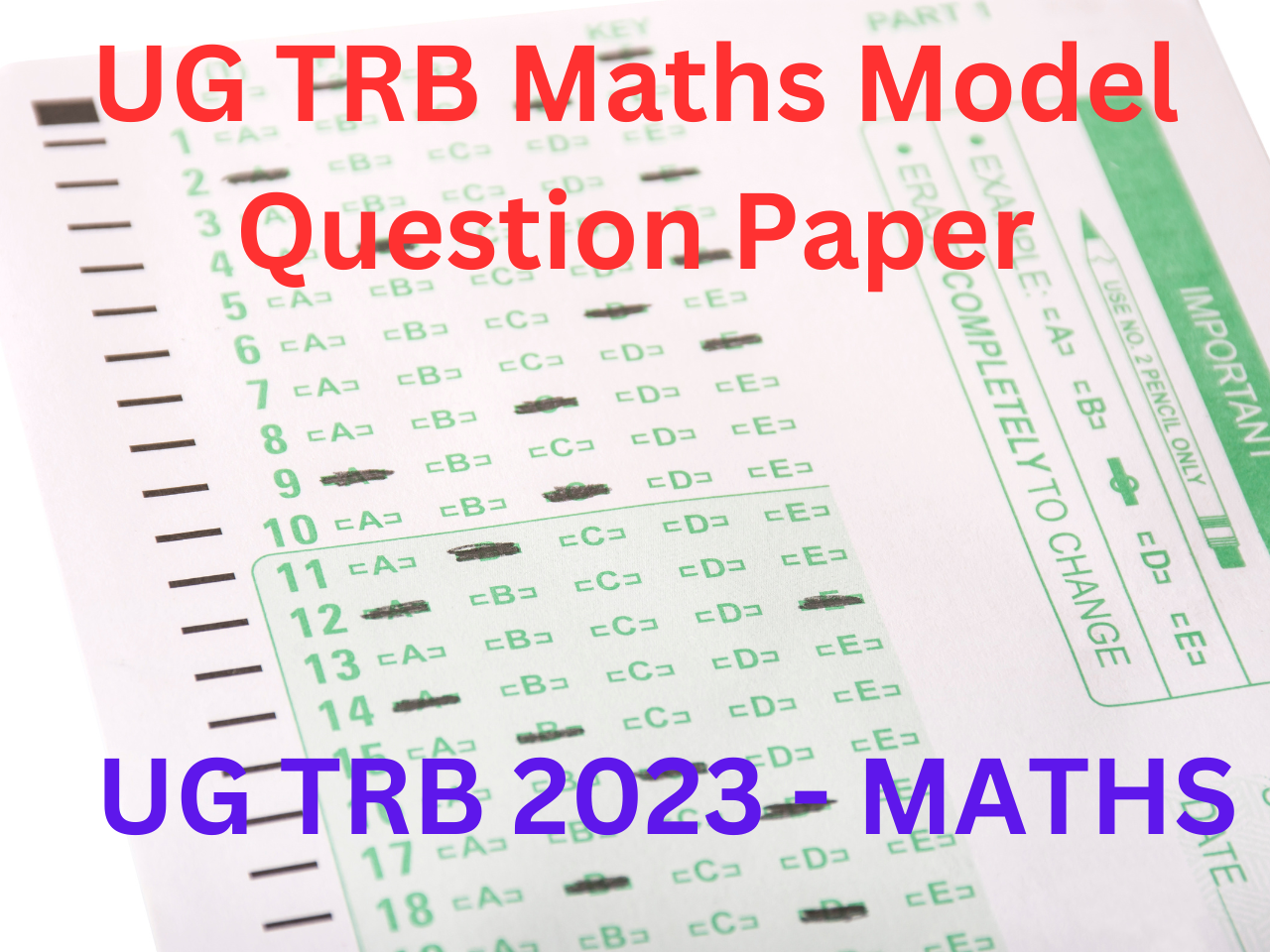# KARKANDU KANITHAM# UG TRB Maths Model Question Paper

## UNIT VIII MECHANICS:

Statics: Forces on a rigid body –Moment of a force – General motion of a rigid body – Equivalent system of forces – Parallel Forces – Forces along the sides of Triangle Couples.

Resultant of several coplanar forces – Equation of line of action of the resultant – Equilibrium of rigid body under three Coplanar forces – Reduction of Coplanar forces into single force and couples – Laws of friction, angle of friction, Equilibrium of a body on a rough inclined plane acted on by several forces – Equilibrium of a uniform Homogeneous string – Catenary – Suspension bridge – Centre of Gravity of uniform rigid bodies.

Dynamics: Velocity and Acceleration – Coplanar motion – Rectilinear motion under constant forces – Acceleration and retardation thrust on a plane – Motion along a Vertical line under gravity – Motion along an inclined plane – motion of connected particles – Newton’s Laws of motion.

Work, Energy and power – Work – Conservative field of force – Power –Rectilinear motion under varying force Simple Harmonic Motion (S.H.M) – S.H.M along a horizontal line – S.H.M along a Vertical line – Motion under gravity in a resisting medium.

Path of a projectile – Particle projected on an inclined plane – Analysis of forces acting on particles and rigid bodies on static equilibrium, equivalent systems of forces, friction, centroids and moments of inertia – Elastic Medium, Impact – Impulsive force – Impact of sphere – Impact of two smooth spheres – Impact of two spheres of two smooth sphere on a plane – oblique impact of two smooth spheres.

Circular motion – Conical Pendulum motion of a cyclist on circular path – Circular motion on a vertical plane – relative rest in revolving cone – simple pendulum – Central Orbits – Conic as Centered Orbit – Moment of inertia

UG TRB 2024 MATHS STUDY MATERIALS ARE AVAILABLE NOW.

FOR DETAILS CONTACT: 9486136884

UG TRB 2023 MATHS